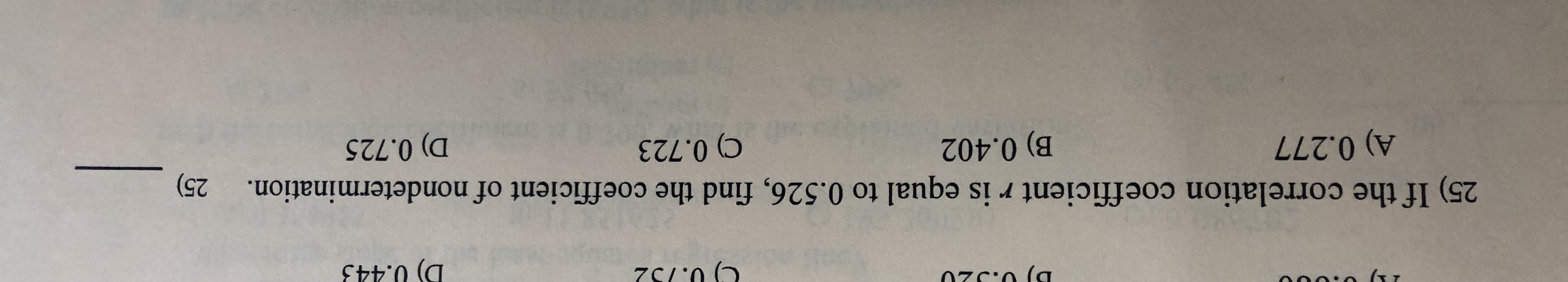# D) 0.443zC/'n25) If the correlation coefficient r is equal to 0.526, find the coefficient of nondetermination.25)A) 0.277B) 0.4029 0.723D) 0.725

Question
30 viewshelp_outlineImage TranscriptioncloseD) 0.443 zC/'n 25) If the correlation coefficient r is equal to 0.526, find the coefficient of nondetermination. 25) A) 0.277 B) 0.402 9 0.723 D) 0.725 fullscreen
check_circle

Step 1

Coefficient of determination:

It also referred as R-square. The coefficient of determination is the squared value of the correlation coefficient. Therefore, it lies between 0 and 1. It can be denoted as R2. The coefficient of variation gives the information about the percentage of variance explained by one with other variable.

Step 2

The coefficient of determination is obtained below:

From the given informat...

### Want to see the full answer?

See Solution

#### Want to see this answer and more?

Solutions are written by subject experts who are available 24/7. Questions are typically answered within 1 hour.*

See Solution
*Response times may vary by subject and question.
Tagged in

### Other